• PhotoShop 图像处理 算法 汇总 置顶2015年01月15日 17:41:34 阅读数：7104 不定期更新 ...... 直接点标题即可链接到原文。 OpenCV 版：OpenCV 图像处理   图层混合算法：   PS图层混合算法之一（不透明度，...

PhotoShop 图像处理 算法 汇总

置顶2015年01月15日 17:41:34
阅读数：7104

不定期更新 ...... 直接点标题即可链接到原文。
OpenCV 版：OpenCV 图像处理

图层混合算法：

PS图层混合算法之一（不透明度，正片叠底，颜色加深，颜色减淡）PS图层混合算法之二（线性加深，线性减淡，变亮，变暗）PS图层混合算法之三（滤色， 叠加， 柔光， 强光）PS图层混合算法之四（亮光， 点光， 线性光， 实色混合）PS图层混合算法之五（饱和度，色相，颜色，亮度）PS图层混合算法之六（差值，溶解， 排除）

图像调整算法：

色彩转换——RGB & HSV色彩转换——RGB & HSL色彩转换——RGB & HSI图像的基本运算——scale， rotation， translationPS 图像调整算法——黑白PS 图像调整算法——自动色阶 （Auto Levels）PS 图像调整算法——亮度调整PS 图像调整算法——自动对比度 （Auto Contrast）PS 图像调整算法——饱和度调整PS 图像调整算法——反相PS 图像调整算法——阈值PS 图像调整算法——色调分离PS 图像调整算法——渐变映射PS 图像特效算法——渐变PS 图像特效算法——百叶窗PS 图像特效算法——镜像渐隐PS 色调——老照片效果PS 图像特效——非线性滤波器PS 色调——颜色运算PS 色调— —颜色梯度图像特效——摩尔纹 moirPS色调— —通道混合

PS 滤镜算法：

PS 滤镜算法原理——高反差保留 （High Pass）

PS 滤镜算法原理——染色玻璃

PS 滤镜算法原理——碎片效果

PS 滤镜算法原理——浮雕效果

PS 滤镜算法原理——照亮边缘

PS 滤镜算法原理——马赛克

PS 滤镜算法原理——曝光过度

PS 滤镜算法原理——拼贴

PS 滤镜——素描算法（一）

PS 滤镜——素描算法（二）

PS 滤镜——运动模糊

PS 滤镜——扩散特效， 毛玻璃 效果

PS 滤镜——径向模糊

PS 滤镜——旋转模糊

PS 滤镜——极坐标变换到平面坐标
PS 滤镜——（扭曲）球面化 Spherize

PS 滤镜——（扭曲）逆球面化（凹陷效果）
PS 滤镜——波浪 wave
PS 滤镜——漩涡 vortex
利用Perlin nosie 完成（PS 滤镜—— 分成云彩）
PS 滤镜— — sparkle 效果
PS 滤镜— — 镜头光晕PS 滤镜— —图像偏移
PS 滤镜— — 万花筒效果
PS 滤镜— —Marble 效果
PS 滤镜— —扇形warp
PS 滤镜— —Twirl Filter
PS滤镜— —波浪效果


展开全文• 一些基本数字图像处理算法 所有的图像算法都在DIPAlgorithm类，并且所有算法都为抽象成员函数。我已经按照java注释规范为所有方法添加使用说明注释，具体实现可见于DIPAlgorithm.java，这里只做算法说明。 1 图像...
一些基本数字图像处理算法
版权声明：本文为原创文章，未经博主允许不得用于商业用途。
所有的图像算法都在DIPAlgorithm类中，并且所有算法都为抽象成员函数。我已经按照java注释规范为所有方法添加使用说明注释，具体实现可见于DIPAlgorithm.java，这里只做算法说明。
1 图像扭曲模仿PS的扭曲功能，通过建立一个三角形映射网格实现对图像的扭曲。
如上图，一共设置了45个控制点围成74个三角形网格
扭曲即形变处理其实是寻找一个函数，以所有网格顶点原始坐标为输入，扭曲后所有网格顶点坐标为输出。为了简化计算任务，采用控制栅格插值方法，对每个三角网格独立计算映射关系，如下图：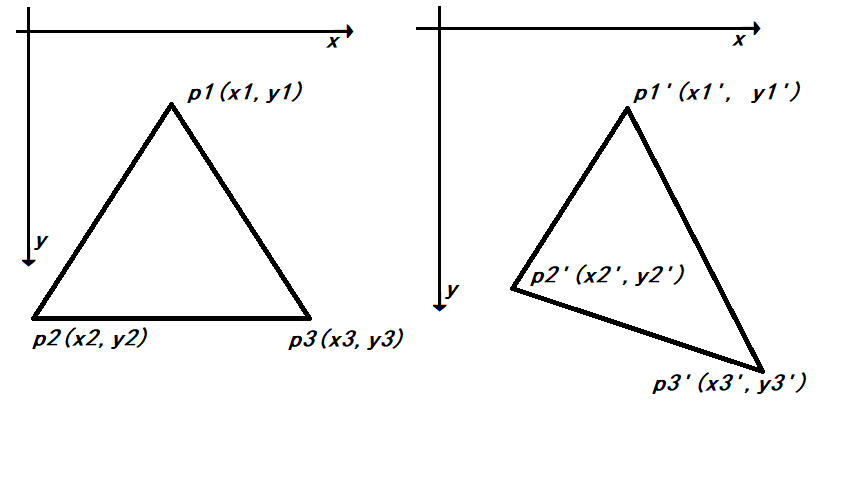即求解矩阵$M$满足$MA = B$，其中$A$为原顶点的齐次矩阵：
$A = \begin{bmatrix} x_{1} & y_{1} & 1 \\ x_{2} & y_{2} & 1 \\ x_{3} & y_{3} & 1 \\ \end{bmatrix}$
B为形变后顶点的其次矩阵：
$B = \lbrack\begin{matrix} x_{1}^{'} & x_{2}^{'} & x_{3}^{'} \\ y_{1}^{'} & y_{2}^{'} & y_{3}^{'} \\ \end{matrix}\rbrack$
M即为$2 \times 3$的映射矩阵，且由于三角形三点不共线，因此A为可逆阵，
$M = BA^{- 1}$
对于三角形中的点$p\left( x,\ y \right)$，其映射后坐标$p^{'} = M\begin{bmatrix} x \\ y \\ 1 \\ \end{bmatrix}$
2 直方图计算
直方图计算实际上即求图像的概率密度函数PDF，只需遍历一次所有像素点即可获得。
3 直方图均衡化算法
对于连续图像直方图均衡化其实是种点运算f,
对不同灰度值做映射，使得所有像素频率相等。
对于点运算f，有如下性质：
$D_{B} = f\left( D_{A} \right),\ H_{B}\left( D_{B} \right)\Delta D_{B} = H_{A}\left( D_{A} \right)\Delta D_{A}$
其中D为灰度值，H即为灰度值在图像中的频数，整理可得
$H_{B}\left( D_{B} \right) = \frac{H_{A}\left( D_{A} \right)\Delta D_{A}}{\Delta D_{B}} = \frac{H_{A}\left( D_{A} \right)}{\frac{\Delta D_{B}}{\Delta D_{A}}} = \frac{H_{A}\left( D_{A} \right)}{\frac{dD_{B}}{dD_{A}}}$
$= \frac{H_{A}\left( D_{A} \right)}{f'(D_{A})} = \frac{H_{A}\left( f^{- 1}\left( D_{B} \right) \right)}{f'(f^{- 1}(D_{B}))}$
即：
寻找函数f使得$H_{B}(D)$为常数$\frac{A_{0}}{D_{m}},A_{0},D_{m}$。
由(1)可知，KaTeX parse error: Undefined control sequence: \
at position 58: …\right)}{f'(D)}\̲
̲\Rightarrow f^{…
即$f\left( D \right) = D_{m}CDF(D)$，CDF即累积分布函数
因此只需求得直方图的前序和即可获得映射关系。
4 图像灰度化
目前比较符合人眼的灰度化权重为0.299、0.578和0.114，为了加速计算使用近似公式$D = (3r + g + 6b)/10$
5 图像二值化
我使用的二值化算法为OSTU大律二值化算法。二值化操作即利用分割阈值u，将图片分为前景后景两部分。OSTU大律法认为使得前景像素和背景像素灰度方差g最大的阈值即为最佳分割阈值。
$g = w_{0}w_{1}\left( u_{0} - u_{1} \right)^{2}$
其中$w_{0},\ w_{1}$为前景、后景在图像中的比例，KaTeX parse error: Undefined control sequence: \
at position 7: u_{0},\̲
̲u_{1}为前景、后景的平均灰度。
在实现时，只需遍历所有灰度，利用CDF求出每种灰度的方差，取最大者作为阈值即可。
6 前景分离
目前主流的前景分离为深度学习算法。这里只使用了最基本的阈值分离法，分别为RGB三个通道设置不同阈值，将小于阈值的像素作为背景，大于阈值的作为前景。
7 滤波
我使用的滤波方法是高斯滤波和中值滤波，高斯滤波即使用二维高斯函数作为滤波函数，中值滤波即使用邻域的中位数作为滤波函数。
高斯滤波器为线性滤波器，可以有效消除高斯噪声。由于高斯函数离中值越近权重越大，因此相对于均值滤波器更加柔和，对边缘的保留效果更好。这里我使用的是如下矩阵做卷积：
$\begin{bmatrix} 1 & 2 & 3 & 2 & 1 \\ 2 & 4 & 6 & 4 & 2 \\ 3 & 6 & 7 & 6 & 3 \\ 2 & 4 & 6 & 4 & 2 \\ 1 & 2 & 3 & 2 & 1 \\ \end{bmatrix}$
中值滤波器为非线性滤波器，可以有效的去除椒盐噪声和斑点噪声并且不会使图像变模糊。
8 形态学扩张和腐蚀
形态学腐蚀可记为$\text{AΘB}$，其中A为输入图像，B为结构单元。对于二值图像，当且仅当当前像素点满足腐结构单元时才会被保留。对于灰度图像，则可类比为最小值，即
$f\Theta b\left( x,y \right) = min\{ f\left( x - x^{'},\ y - y^{'} \right) - b(x^{'},y')|(x^{'},y^{'} \in D_{b})\}$
形态学扩张可看作腐蚀的逆操作,记作$A\bigoplus B$，对于二值图像，将每个有效像素点的邻域结构单元置1，对于灰度图像则取最大值，即
$f\bigoplus b\left( x,y \right) = max\{ f\left( x - x^{'},\ y - y^{'} \right) - b(x^{'},y')|(x^{'},y^{'} \in D_{b})\}$
本程序将结构单元b统一设定为5*5矩形。
通过扩张和腐蚀的结合可实现结构开运算（$AoB = \left( \text{AΘB} \right)\bigoplus B$）和结构闭运算（$AoB = \left( A\bigoplus B \right)\text{ΘB}$）对图像进行粗化、细化、滤波等处理
9 傅里叶变换和滤波
变换公式
傅里叶变换可以将信号从时域转换到频域，因此可以看出许多时域中不明显的特征。二维傅里叶变换（CFT）公式如下：
$F\left( u,v \right) = \iint_{}^{}{f\left( x,y \right)e^{- 2\pi\overrightarrow{j}(ux + vy)}}\text{dxdy}$
其中${\overrightarrow{j}}^{2} = - 1,f,F$，同样二维傅里叶逆变换公式如下:
$f\left( x,y \right) = \iint_{}^{}{F\left( u,v \right)e^{2\pi\overrightarrow{j}(ux + vy)}}\text{dudv}$
对于离散函数，可以定义离散二维傅里叶变换（DFT）和逆变换：
$G\left( m,n \right) = \frac{1}{\sqrt{\text{MN}}}\sum_{\begin{matrix} 0 \leq \ i\ \leq \ M - 1 \\ 0 < k < N - 1\ \\ \end{matrix}}^{}{g\left( i,k \right)e^{- 2\pi\overrightarrow{j}(\frac{\text{im}}{M} + \frac{\text{jn}}{N})}}$
$g\left( i,k \right) = \frac{1}{\sqrt{\text{MN}}}\sum_{\begin{matrix} 0 \leq \ m\ \leq \ M - 1 \\ 0 < n < N - 1\ \\ \end{matrix}}^{}{g\left( m,n \right)e^{2\pi\overrightarrow{j}(\frac{\text{im}}{M} + \frac{\text{jn}}{N})}}$
DFT可以理解为对连续二维信号进行了频率为M,
N的采样，之后通过计算其和频域空间M*N个基向量的相关性（在该方向投影）将时域信号映射到频域。iDFT可以理解为通过M*N个基向量合成原始时域信号。
矩阵表示
傅里叶变换实际上是一种线性变换，因此在实际计算中常常将$g$扩充为$N*N$方阵，此时DFT可以通过矩阵表示：$G = \mathcal{W}^{- 1}g\mathcal{W},\mathcal{W}_{\text{ik}} = \frac{1}{N}e^{2\pi\overrightarrow{j}\frac{\text{ik}}{N}}$。
易知$\mathcal{W}_{\text{ik}} = \mathcal{W}_{\text{ki}}$，且为正交矩阵，因此$\mathcal{W}$为酉矩阵，即$\mathcal{W}^{- 1} = \left( \mathcal{W}^{*} \right)^{T} = \mathcal{W}^{*}$，$G = \mathcal{W}^{*}g\mathcal{W}$，其中$F^{*}F$。
由于傅里叶变换为酉变换，即$\mathcal{W}^{t} = \mathcal{W}^{- 1}$
图像的傅里叶变换
对于二维图片可以看作二维矩阵，因此可以进行DFT。二维图片经过DFT后获得的复矩阵的模矩阵可以表示每个频率信号的强度（也可看作先做自相关后再进行傅里叶变换），经过适当处理即可转化为灰度能量谱图片。
线性噪声在频域中通常为点或线，因此可以通过傅里叶变换后进行滤波再通过逆变换复原图片。
算法实现
在实际实现时，根据欧拉公式，$e^{- \overrightarrow{j}t} = cost - \overrightarrow{j}\text{sint},\ e^{\overrightarrow{j}t} = cost + \overrightarrow{j}\text{sint}$，因此傅里叶变换的核矩阵可以表示为$\mathcal{W}_{\text{ik}} = \frac{\cos\left( 2\pi ik \right) - \overrightarrow{j}\sin\left( 2\pi ik \right)}{N}$，为方便运算将$\mathcal{W}$分解为虚部系数$\mathcal{W}_{\text{lm}}$和实部系数$\mathcal{W}_{\text{re}}$，其中则$\mathcal{W} = \mathcal{W}_{\text{re}} + \overrightarrow{j}\mathcal{W}_{\text{lm}}$。变换结果同样分解为$G = G_{\text{re}} + \overrightarrow{j}G_{\text{lm}}$，则DFT可以表示为:
$G = \mathcal{W}^{*}g\mathcal{W =}\left( \mathcal{W}_{\text{re}} - \overrightarrow{j}\mathcal{W}_{\text{lm}} \right)g\left( \mathcal{W}_{\text{re}} + \overrightarrow{j}\mathcal{W}_{\text{lm}} \right) = \mathcal{W}_{\text{re}}g\mathcal{W}_{\text{re}} + \mathcal{W}_{\text{lm}}g\mathcal{W}_{\text{lm}} - \overrightarrow{j}\left( \mathcal{W}_{\text{lm}}g\mathcal{W}_{\text{re}} + \mathcal{W}_{\text{re}}g\mathcal{W}_{\text{lm}} \right)$
$\left\{ \begin{matrix} G_{\text{re}} = \mathcal{W}_{\text{re}}g\mathcal{W}_{\text{re}} + \mathcal{W}_{\text{lm}}g\mathcal{W}_{\text{lm}} \\ G_{\text{lm}} = - \mathcal{W}_{\text{lm}}g\mathcal{W}_{\text{re}} - \mathcal{W}_{\text{re}}g\mathcal{W}_{\text{lm}} \\ \end{matrix} \right.\$
同理，iDFT可以表示为：
$g = \left( \mathcal{W}_{\text{re}} + \overrightarrow{j}\mathcal{W}_{\text{lm}} \right)(G_{\text{re}} + {\overrightarrow{j}G}_{\text{lm}})\left( \mathcal{W}_{\text{re}} - \overrightarrow{j}\mathcal{W}_{\text{lm}} \right)$
其中，为了将能量谱转化为可见的灰度图，为能量谱取对数值进行归一化。且由于在频域中两个维度频率都为0时（即$\mathcal{W}_{00}$处）为图像能量的总和，因此通过$log(e + 1)*\frac{256}{\log\left( \mathcal{W}_{00} + 1 \right)}$可以做进一步归一化。
算法代码可见github


展开全文数字图像处理
• 在研究的过程，有时候会碰到很多有意思的图像处理算法，算法极具新意，并且能够产生非常有意思的结果。 算法简介 1、图像镶嵌 图像镶嵌也叫图像混合（Image Blending）、图像剪接（Image Editing），是通过特定...


之前见了一些文章讨论了一些有趣的图像处理算法

下面总结列出：

在研究的过程中，有时候会碰到很多有意思的图像处理算法，算法极具新意，并且能够产生非常有意思的结果。

算法简介

1、图像镶嵌

图像镶嵌也叫图像混合（Image Blending）、图像剪接（Image Editing），是通过特定的图像处理方法将本来毫无关系的两幅图无缝剪辑到一起，并能够很好地融合两者之间的剪接处，产生以假乱真的效果，不信我们就来看看。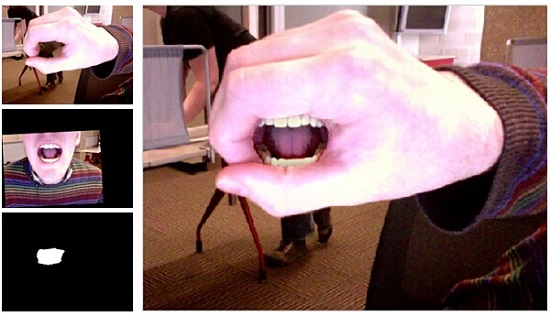上图左边的手和嘴巴在两幅不同的图中，只要给定一个模板，就可以把一幅图中模板指定的部分无缝拼接到另外一幅图中去，从右边的结果来看是不是看上去毫无违和感？比某些人的PS技术强多了。

类似剪接效果的还有下面这种技术，该算法同样也是划定一个区域，能够将两幅图中的指定区域图像都拿出来，拼接到第三幅图上去，结果简直天衣无缝。这两个算法的技术细节可看参考文献。同样也可以参考这位小哥的代码。2、黑白图像着色

家里有黑白的老照片，想把它变成彩色照片，看看老一辈人真实的模样，那你可以试试下面这个图像着色（Colorization）算法。你只要拿彩笔在图像上画几笔，剩下的事情就交给算法啦。这个算法除了给黑白图片着色，还能够给视频着色呢！不信就去文献看看。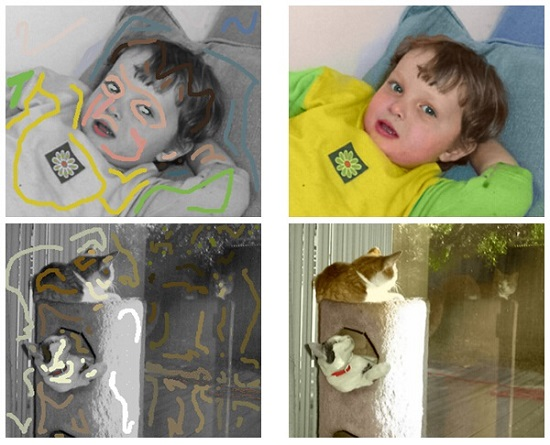3、图像超分辨

还记得小时候的红白机画面么，分辨率很低，如果放大的话，渣渣的马赛克就来了，想不起来了？想想《愤怒的小鸟》那个游戏里面马赛克小鸟，实在让人心塞，如果用了这个算法效果绝对就不一样了。该超分辨算法能够将分辨率非常低的图像提取与分辨率无关的向量，最后的高分辨率图像真是碉堡了，完虐普通的最近邻插值法放大的图像。还有人提供了Python源代码呢。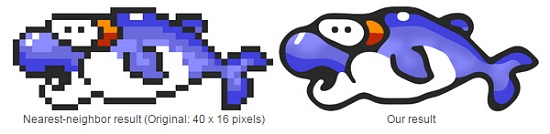4、图像去模糊

拍照片的时候手抖，拍出来的照片模糊了怎么办？可以背个三脚架，或者花钱升级相机，变成防抖的。或者你也可以使用以下的图像处理方法，拍完之后处理，就可以得到清晰的图像了。说不定哪一天珍贵的一瞬间拍模糊了，还能用来救救急。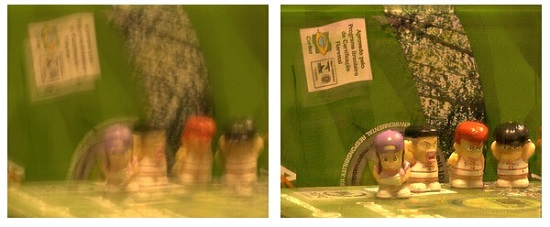5、图像编辑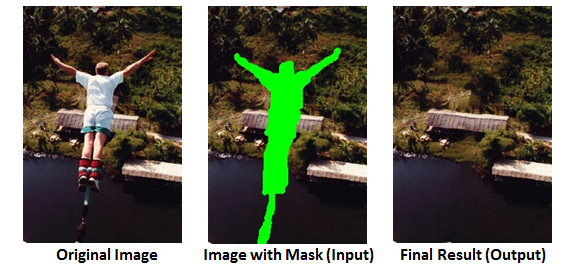如果拍上去的建筑物看上去破破烂烂的，想填补一下这个破洞，或者想干脆在图像中再复制同一个物体，可以试试下面这个方法。顺便说一句，这两个方法都应用到了PhotoShop上了。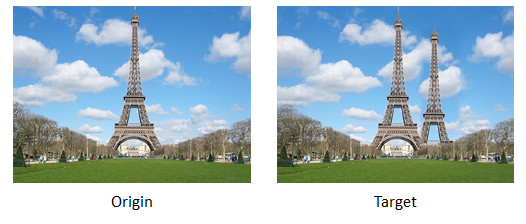参考文献

 Peter J. Burt and Edward H. Adelson. 1983. A multiresolution spline with application to image mosaics. ACM Trans. Graph. 2, 4 (October 1983)

 Patrick Pérez, Michel Gangnet, and Andrew Blake. 2003. Poisson image editing. ACM Trans. Graph. 22, 3 (July 2003)

 Anat Levin, Dani Lischinski, and Yair Weiss. 2004. Colorization using optimization. ACM Trans. Graph. 23, 3 (August
2004)

 Johannes Kopf and Dani Lischinski. 2011. Depixelizing Pixel ArtACM Transactions on Graphics (Proceedings
of SIGGRAPH 2011).

 Xu, Li, and Jiaya Jia. 2010. Two-phase kernel estimation for robust motion deblurring.ECCV.
Springer Berlin Heidelberg, 2010. 157-170.

 Image Completion

 Y. Wexler, E. Shechtman and M. Irani “Space-Time Video Completion” Computer Vision and Pattern
Recognition (CVPR), Washington, June 2004.

 Connelly Barnes, Eli Shechtman, Adam Finkelstein, and Dan B Goldman. 2009.PatchMatch: a randomized
correspondence algorithm for structural image editing. ACM Trans. Graph. 28, 3, Article 24 (July 2009)

 Connelly Barnes, Eli Shechtman, Dan B Goldman, The Generalized PatchMatch Correspondence Algorithm,
ECCV, 2010

还有一篇：

一、像素图生成向量图的算法数字时代早期的图片，分辨率很低。尤其是一些电子游戏的图片，放大后就是一个个像素方块。
Depixelizing（http://research.microsoft.com/en-us/um/people/kopf/pixelart/）算法可以让低分辨率的像素图转化为高质量的向量图。

二、黑白图片的着色算法让老照片自动变成彩色的算法（http://www.cs.huji.ac.il/~yweiss/Colorization/）。

三、消除阴影的算法四、HDR照片的算法所谓”HDR照片”，就是让明亮处变得更亮、让阴暗处变得更暗，从而让照片产生强烈的对比效果。

实现HDR的软件有很多，这里推荐G’MIC（http://gmic.eu/）。它是GIMP图像编辑软件的一个插件，代码全部开源。

五、消除杂物的算法所谓”消除杂物”，就是在照片上划出一块区域，然后用背景自动填补。Resynthesizer可以做到这一点，它也是GIMP的一个插件。

六、自动合成照片的算法根据一张草图，选择原始照片，然后把它们合成在一起，生成新照片（http://www.scriptol.com/design/sketchtophoto.php）。

七、美容算法自动对容貌进行”美化”的算法（http://www.scriptol.com/programming/algorithm-pretty-face.php）

转载：https://blog.csdn.net/qq_26499769/article/details/51816862
展开全文• OpenCV 版：OpenCV 图像处理 图层混合算法PS图层混合算法之一（不透明度，正片叠底，颜色加深，颜色减淡）PS图层混合算法之二（线性加深，线性减淡，变亮，变暗）PS图层混合算法之三（滤色， 叠加， 柔光， ...
OpenCV 版：OpenCV 图像处理

PS图层混合算法之一（不透明度，正片叠底，颜色加深，颜色减淡）PS图层混合算法之二（线性加深，线性减淡，变亮，变暗）PS图层混合算法之三（滤色， 叠加， 柔光， 强光）PS图层混合算法之四（亮光， 点光， 线性光， 实色混合）PS图层混合算法之五（饱和度，色相，颜色，亮度）PS图层混合算法之六（差值，溶解， 排除）

图像调整算法：

色彩转换——RGB & HSV色彩转换——RGB & HSL色彩转换——RGB & HSI图像的基本运算——scale， rotation， translationPS 图像调整算法——黑白PS 图像调整算法——自动色阶 （Auto Levels）
PS 图像调整算法——亮度调整
PS 图像调整算法——自动对比度 （Auto Contrast）
PS 图像调整算法——饱和度调整
PS 图像调整算法——反相
PS 图像调整算法——阈值
PS 图像调整算法——色调分离PS 图像调整算法——渐变映射PS 图像特效算法——渐变
PS 图像特效算法——百叶窗
PS 图像特效算法——镜像渐隐PS 色调——老照片效果PS 图像特效——非线性滤波器PS 色调——颜色运算PS 色调— —颜色梯度图像特效——摩尔纹 moirPS色调— —通道混合

PS 滤镜算法：

PS 滤镜算法原理——高反差保留 （High Pass）

PS 滤镜算法原理——染色玻璃

PS 滤镜算法原理——碎片效果

PS 滤镜算法原理——浮雕效果

PS 滤镜算法原理——照亮边缘

PS 滤镜算法原理——马赛克

PS 滤镜算法原理——曝光过度

PS 滤镜算法原理——拼贴

PS 滤镜——素描算法（一）

PS 滤镜——素描算法（二）

PS 滤镜——运动模糊

PS 滤镜——扩散特效， 毛玻璃 效果

PS 滤镜——径向模糊

PS 滤镜——旋转模糊

PS 滤镜——极坐标变换到平面坐标

PS 滤镜——（扭曲）球面化 Spherize

PS 滤镜——（扭曲）逆球面化（凹陷效果）

PS 滤镜——波浪 wave

PS 滤镜——漩涡 vortex

利用Perlin nosie 完成（PS 滤镜—— 分成云彩）

PS 滤镜— — sparkle 效果

PS 滤镜— — 镜头光晕

PS 滤镜— —图像偏移

PS 滤镜— — 万花筒效果

PS 滤镜— —Marble 效果

PS 滤镜— —扇形warp

PS 滤镜— —Twirl Filter

PS滤镜— —波浪效果

展开全文photoshop 算法 图像处理
• 图像USM锐化是图像处理软件常见的功能，而PHOTOSHOP的USM锐化相对而言效果较好，网上有很多揭秘PhotoShop USM锐化算法的文章，但是，算法效果与PS相比，差距较大，今天本人针对PS的USM锐化，给出一个效果比较...
    图像USM锐化是图像处理软件中常见的功能，而PHOTOSHOP中的USM锐化相对而言效果较好，网上有很多揭秘PhotoShop USM锐化算法的文章，但是，算法效果与PS相比，差距较大，今天本人针对PS的USM锐化，给出一个效果比较接近的算法，跟大家分享一下，有助于大家走出误区。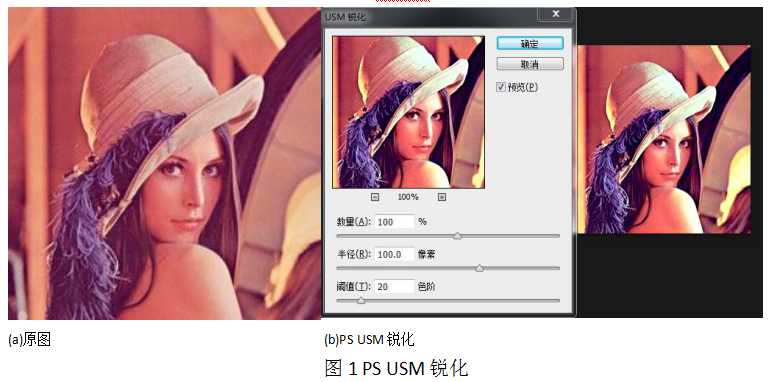网络中流行的算法(来自Imageshop)，连接如下：Imageshop USM

算法过程如下：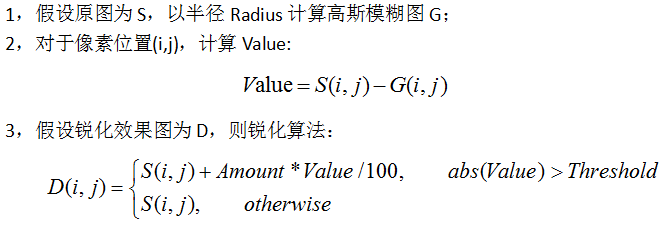以Lena测试图为例，使用Imgeshop提供的DEMO测试如下图2：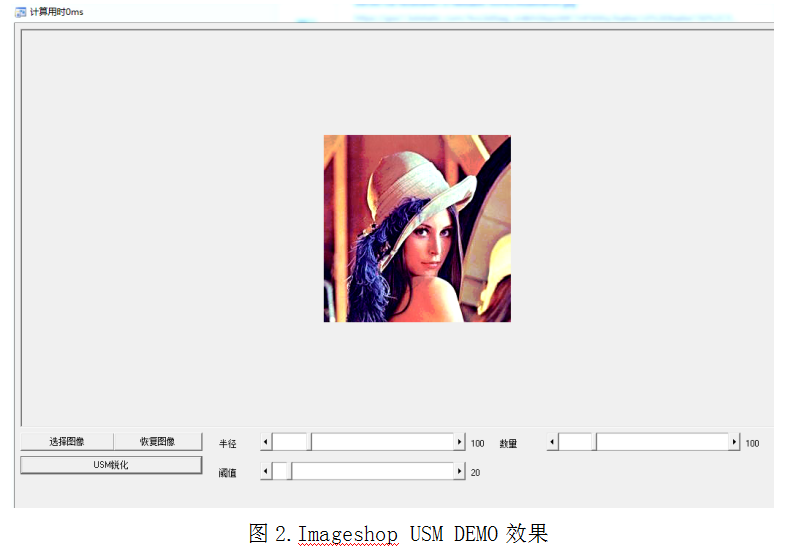对比PS如下图3：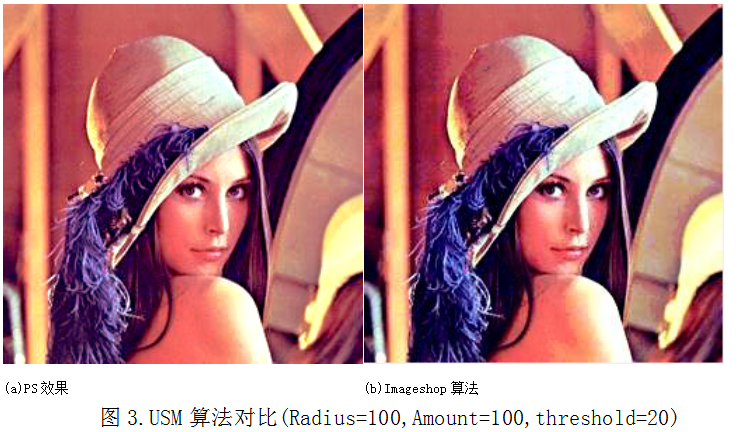问题所在如下图4所示：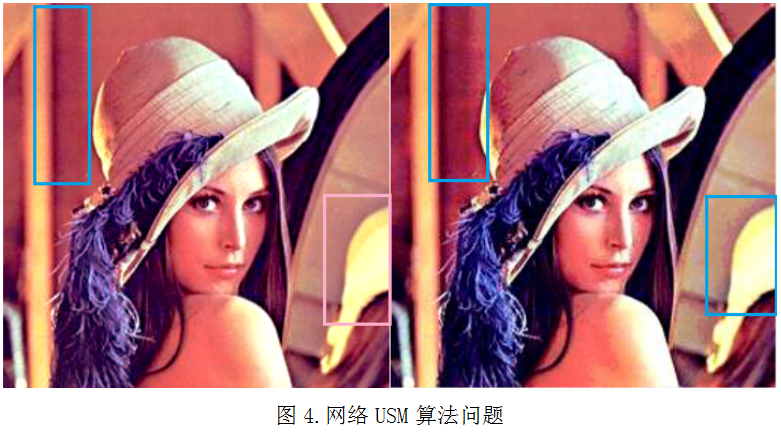如图4中所示，在蓝色标记区域，imageshop所提算法存在块状不连续问题，而PS算法非常自然，过渡平滑。

造成这个问题的原因是在算法3中我们使用了一个简单的阈值Threshold，将图像锐化与非锐化区域分成了两类，两类之间没有过渡，所以出现了这个现象。

这里给出本人的算法：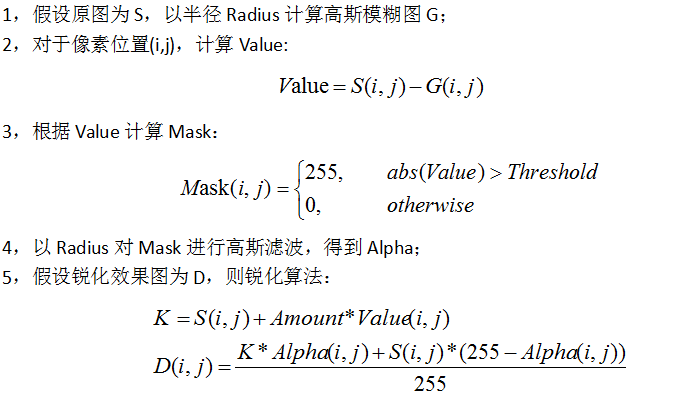代码实现如下：

int f_USM(unsigned char* srcData,int width, int height,int stride,int radius, int amount, int threshold)
{
int ret = 0;
return ret;
amount = CLIP3(amount, 0,500);
threshold = CLIP3(threshold, 0,255);
unsigned char* gaussData = (unsigned char*)malloc(sizeof(unsigned char) * height * stride);
memcpy(gaussData, srcData, sizeof(unsigned char) * height * stride);
int i, j, r, g, b, offset;
offset = stride - width * 3;
amount = amount * 128 / 100;
unsigned char* pSrc = srcData;
unsigned char* pDst = gaussData;
unsigned char* maskData = (unsigned char*)malloc(sizeof(unsigned char) * height * stride);
for(j = 0; j < height; j++)
{
for(i = 0; i < width; i++)
{
pMask = abs(pSrc - pDst) < threshold ? 0 : 128;
pMask = abs(pSrc - pDst) < threshold ? 0 : 128;
pMask = abs(pSrc - pDst) < threshold ? 0 : 128;
pDst += 3;
pSrc += 3;
}
pDst += offset;
pSrc += offset;
}
pDst = gaussData;
pSrc = srcData;
for(j = 0; j < height; j++)
{
for(i = 0; i < width; i++)
{
b = pSrc - pDst;
g = pSrc - pDst;
r = pSrc - pDst;

b = (pSrc + ((b * amount) >> 7));
g = (pSrc + ((g * amount) >> 7));
r = (pSrc + ((r * amount) >> 7));

b = (b * pMask + pSrc * (128 - pMask)) >> 7;
g = (g * pMask + pSrc * (128 - pMask)) >> 7;
r = (r * pMask + pSrc * (128 - pMask)) >> 7;

pSrc = CLIP3(b, 0, 255);
pSrc = CLIP3(g, 0, 255);
pSrc = CLIP3(r, 0, 255);
pSrc += 3;
pDst += 3;
}
pSrc += offset;
pDst += offset;
}
free(gaussData);
return ret;
};

本文算法的效果如下图5：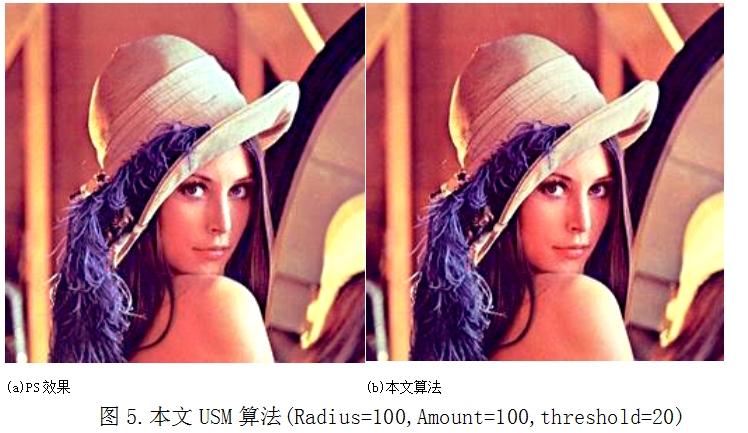在图5中，我们可以看到，之前蓝色区域的像素过渡平滑自然，跟PS效果接近。

上述内容是本人对PS USM锐化算法的一些研究，主要针对网络中比较流行的USM算法进行了对比分析，算法改进，以得到更接近于PS USM锐化的算法，跟大家分享一下！

本人QQ1358009172，微信公众号:SF图像算法
展开全文• 该系列文章是讲解Python OpenCV图像处理知识，前期主要讲解图像入门、OpenCV基础用法，中期讲解图像处理的各种算法，包括图像锐化算子、图像增强技术、图像分割等，后期结合深度学习研究图像识别、图像分类应用。...Python 图像处理 OpenCV
• 一些我学习数字图像处理中的心得和建议，欢迎有兴趣的朋友共同参与讨论。工欲善其事，必先利其器。有时一些方法论之类的东西往往能让你少走很多弯路图像处理 学习方法
• 1)将256*256分辨率的图像变为128*128分辨率可以将源图像划分成2*2的子图像块,然后将2*2的 子图像块的所有像素颜色均按照F(i,j)的颜色值进行设定，达到降低分辨率的目的。 如: F(i,j) F(i,j+1) F(i,j) F(i,j)...
• 程序员拿到设计师的滤镜设计稿...这一点多多少少还是有些挑战，因为ps经过多年发展后，不同版本有些功能效果还不一样，比如最为常见的亮度、对比度调节，算法很简单，但是要做到和ps效果一样，还是要花时间摸索、调试。图像处理 滤镜
• 1、图像处理的基础：冈萨雷斯的《数字图像处理》这本书必看。里面算法虽然老，但是还是很实用的。搭配它拍套的《数字图像处理的Matlab实现》方便Matlab实现。 2、机器学习相关的，机器学习的常用算法最好都知道，...图像 图像算法 图像算法工程师图像处理 算法 颜色空间 photoshop
• 前言：之前在公司做项目的用到photoshop颜色空间的一些相关方法，在此总结一下。下面原理部分是从文档里截取来的。需要复制的童鞋自己手写一下~ 2、程序部分 1）Matlab实验程序图像处理 photoshop 算法 颜色空间
• 图像的饱和度调整有很多方法，最简单的就是判断每个象素的R、G、B值是否大于或小于128，大于加上调整值，小于则减去调整值；也可将象素RGB转换为HSV或者HSL，然后调整其S部分，从而达到线性调整图象饱和度的目的。这...算法
• 前言：之前在公司做项目的用到photoshop颜色空间的一些相关方法，在此总结一下。下面原理部分是从我的总结文档里截取来的。需要复制的童鞋自己手写一下~ 2、程序部分 1）Matlab实验程序。...R=doubphotoshop 图像处理 算法 颜色空间
• 就说ps、天天P图、美图秀秀、可牛等这些每个软件，有好多个功能都要用到图像变形，比如瘦脸眼睛放大、图像方向校正、图像全景等，在我的另外一篇博文全景矩形还原，就要用到图像变形算法。 可以说ps中的一些图像扭曲...
• 因为图像处理算法多是针对矩阵，所以实现和调试比较快。 PS:2009.10.27这是我博客最烂的帖子，但是却有如此多的访问量，唉。其实算法的代码是不可能公开的，因为算法的关键部分申请了软件著作权的。不过，其基础...算法 图像处理
...